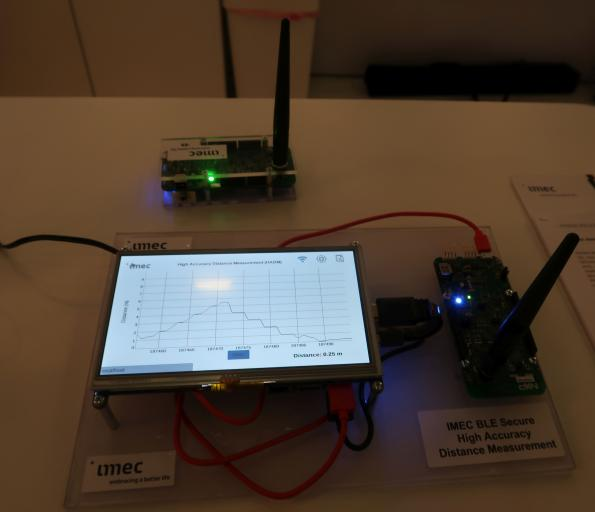# BLE HADM Basic and Usage for Keyless Entry

Make it to the Right and Larger Audience

Blog

# BLE HADM Basic and Usage for Keyless Entry

In Nov 2018, Belgium IMEC demonstrated the first solution for secure and very accurate passive keyless car entry based on Bluetooth Low Energy (BLE) technology.This technology enables a seamless car access experience where a BLE device, such as a mobile phone, acts as a digital car key. Imec’s demonstrator builds on the research center’s secure and very high accuracy ranging algorithms implemented on BLE. Imec’s BLE ranging technology achieves an accuracy better than 30 centimeters – which is over 10 times better than current approaches, even those already using phase-based ranging techniques – and will be enriched with physical layer security features that create a secure distance measurement between two Bluetooth-enabled communication devicesThis phase based ranging technology is adopted by Bluetooth and is known as HADM, High Accuracy Distance Measurement.

In a nutshell, HADM calculates the distance between two points, A and B, from the single wave or single tone phase difference. Let’s say A sends a single tone of frequency f to B and the distance between A and B is L. Then point B sees the phase of the received single tone as$\phi_{A2B}=(2\pi f L / c + \phi_0) mod 2\pi$

where c is light speed and$\phi_0$ is the initial phase difference between A’s LO and B’s LO. (LO is RF local oscillator)

Point B is required to bounce back a single tone of the same frequency and the received phase. Point A receives this single tone and sees the phase as$\phi_{RT}=(2\pi f L / c - \phi_0 + \phi_{A2B}) mod 2\pi$

where$\phi_{RT}$ refers to Round Trip (RT) total phase difference. Therefore we have$\phi_{RT}=(4\pi f L / c ) mod 2\pi$

Above equation means value of$\phi_{RT}$ repeats itself every half wavelength, c/f. BLE frequency is around 2.4Ghz so wavelength is about 12cm. Then phase repeats every 6cm is obviously not very useful. However, if we do the same operation with two frequencies, f1 and f2, we have$\phi_{RT}(f_1)=(4\pi f_1 L / c ) mod 2\pi$$\phi_{RT}(f_2)=(4\pi f_2 L / c ) mod 2\pi$

and further$\phi_{RT}(\Delta f)=(4\pi (f_2-f_1) L / c ) mod 2\pi$

If$\Delta f = f_2 -f_1$ is 1Mhz, then above equation can make value of$\phi_{RT}(\Delta f)$ repeat itself around every 80 meters. It is good enough for short range distance measurement.

To improve measurement accuracy and combat channel fading, more than two frequencies can be used$\phi_{RT}(\Delta f)=\Sigma^{\Delta f}(4\pi \Delta f L / c ) mod 2\pi$

1. A and B communicates and initiates HADM
2. A sends single tone of frequency f to B for a pre-arranged time
3. B does frequency and phase measurement
4. B sends back single tone of same frequency and measured phase for a pre-arranged time
5. A does frequency and phase measurement. A has RT phase difference of one frequency f.

Repeat 1-5 for multiple frequencies.

Normal BLE packets are used for channel control and management. BTW, to send and receive single tone in BLE is nothing new. Single tone is already adopted in BLE test mode.Author brief is empty
Groups:

## IoT and Smart DevicesWireless

Tags:

1.chieuluu 6 months ago
0
-0

Good doc

4
2.minhlu 7 months ago
0
-0

30cm could be good enough to open many applications

5
3.0
-0

Where does that 30cm accuracy come from?

4
4.jieFan 11 months ago
0
-0

Nice short post.

Bluetooth SIG is doing the right thing and keeps adding market driven new stuff. They want to include everthing can be done at 2.4G low powe radio into it. Sometimes they do miss here and there. Hope hadm is not a flop as AoA and AoD.

5
•minhlu 7 months ago
0
-0

what is aoa and aod?

0